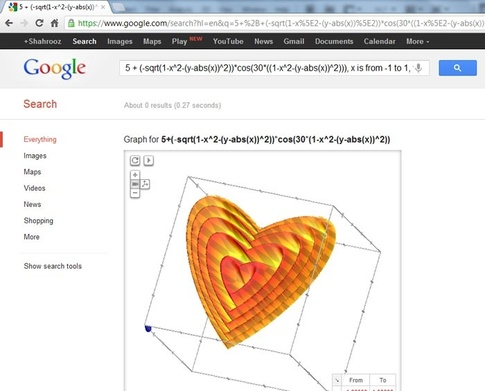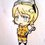# Best Math graphs?

Hey Brillianticians!

without long talks , this discussion is about the best and the most beautiful math graphs, do you know any function, equation, implicit function have a fascinating graph put it here.

i will start with this:its implicit function is $5+(-\sqrt{1-x^2-(y-|x|)^2)}\cos (30((1-x^2-(y-|x|)^2)))$Note by Abdeslem Smahi
4 years, 6 months ago

This discussion board is a place to discuss our Daily Challenges and the math and science related to those challenges. Explanations are more than just a solution — they should explain the steps and thinking strategies that you used to obtain the solution. Comments should further the discussion of math and science.

When posting on Brilliant:

• Use the emojis to react to an explanation, whether you're congratulating a job well done , or just really confused .
• Ask specific questions about the challenge or the steps in somebody's explanation. Well-posed questions can add a lot to the discussion, but posting "I don't understand!" doesn't help anyone.
• Try to contribute something new to the discussion, whether it is an extension, generalization or other idea related to the challenge.

MarkdownAppears as
*italics* or _italics_ italics
**bold** or __bold__ bold
- bulleted- list
• bulleted
• list
1. numbered2. list
1. numbered
2. list
Note: you must add a full line of space before and after lists for them to show up correctly
paragraph 1paragraph 2

paragraph 1

paragraph 2

[example link](https://brilliant.org)example link
> This is a quote
This is a quote
    # I indented these lines
# 4 spaces, and now they show
# up as a code block.

print "hello world"
# I indented these lines
# 4 spaces, and now they show
# up as a code block.

print "hello world"
MathAppears as
Remember to wrap math in $$ ... $$ or $ ... $ to ensure proper formatting.
2 \times 3 $2 \times 3$
2^{34} $2^{34}$
a_{i-1} $a_{i-1}$
\frac{2}{3} $\frac{2}{3}$
\sqrt{2} $\sqrt{2}$
\sum_{i=1}^3 $\sum_{i=1}^3$
\sin \theta $\sin \theta$
\boxed{123} $\boxed{123}$

Sort by:

Graph 2

Graph 3

Graph 4

Graph 5

Graph 6

Graph 7

Graph 8

Graph 9

I sure did a lot of random stuff back then

- 4 years, 6 months ago

That's really amazing!

- 4 years, 6 months ago

Wow!! How did you think of such things? You could make an art gallery of graphs ;)

- 4 years, 6 months ago

$[(x-1)^2 + y^2 - 4] [(x+1)^2 + y^2 - 4] [x^2 + (y - \sqrt [ 2 ]{ 3 } )^2 - 4] < c$

For $c=1$For $c=6$For $c=12$- 4 years, 6 months ago

LOL. The last one looks like Shinchan.

- 4 years, 6 months ago

- 4 years, 6 months ago- 4 years, 6 months ago

Wow! It's nice. Loved it.

- 4 years, 6 months ago